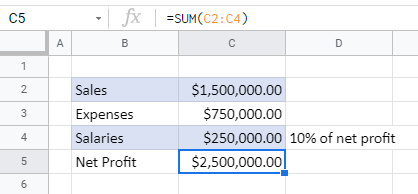# How to Allow Circular References in Excel & Google Sheets

This tutorial demonstrates how to allow circular references in Excel and Google Sheets.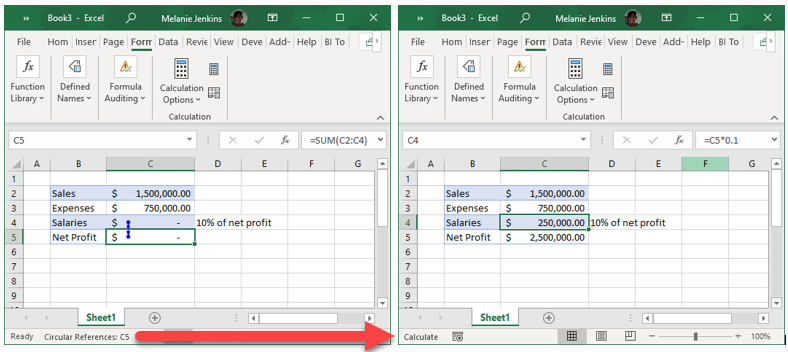By default, Excel returns an error message when a circular reference is detected, and the formula causing the circular reference isn’t calculated. On some occasions, you may need to allow the use of circular references in Excel.

## Enable Iterative Calculation

1. In the Ribbon, go to File > Options > Formulas.
2. Check Enable iterative calculation and set the Maximum Iterations and Maximum Change, or leave at the default values of 100 and 0.001.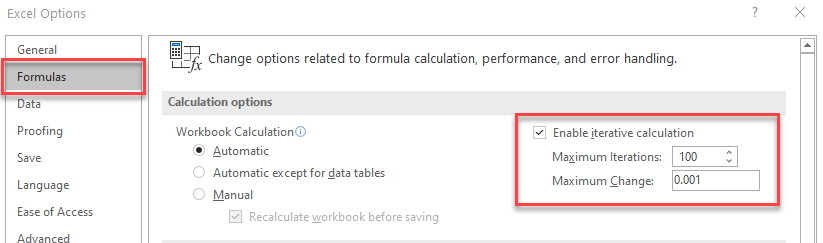Note: Maximum Iterations is the number of times that Excel will repeat the calculation until it stops (i.e., 100). Maximum Change is the maximum difference allowed between the iterations of the calculation. If the maximum change is reached, Excel will stop repeating the calculation even if the number of times it has recalculate has not reached maximum iterations.

## Allow Circular References in Google Sheets

Google Sheets also shows an error when a circular reference is detected.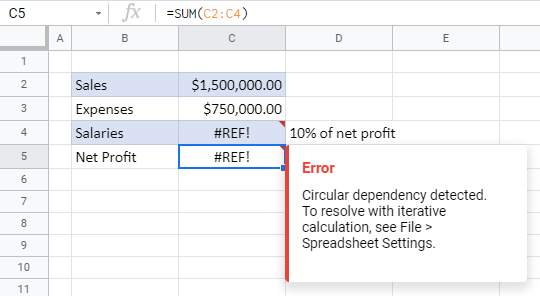1. To allow circular references, in the Menu, go to File > Spreadsheet settings.
2. Select the Calculation tab, and then change the Iterative calculation in the drop down to On.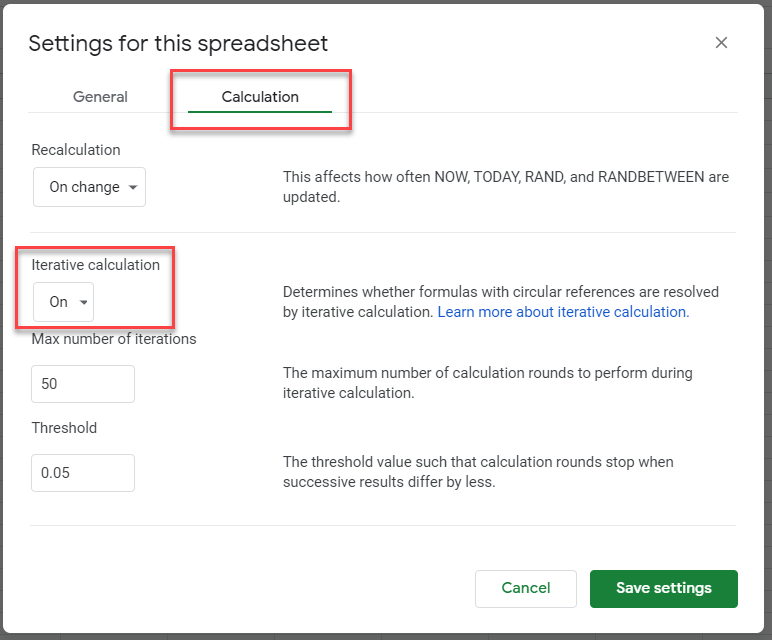1. Adjust the Max number of iterations and Threshold if needed, and then click Save settings.